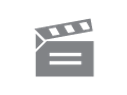Media not available in the Digital Archive
Description
This programme defines congruency in non-Euclidean geometry and then proves that the area of a triangle in this space is proportioned to pi minus the angle sum of the triangle.
Module code and title: M203, Introduction to pure mathematics M203; 22 24-07-1981 1981 00:22:55 + Show more... David Saunders Jeremy Gray; Allan I.,1936-2013 Solomon BBC Open University Euclidean; Kaleidoscope; Non-Euclidean reflections; Geometric transformation Geometry, Non-Euclidean Allan Solomon introduces the programme by briefly recapping on the nature of non-Euclidean geometry as depicted in M101. Jeremy Gray demonstrates these principles with simple drawings, showing that there are an infinite number of parallel lines through a given point to a given line. He then discusses the problem of copying triangles within the non Euclidean world. He argues that what is needed to resolve this difficulty is a definition of allowable transformations in non-Euclidean geometry. Using computer graphics he considers reflection in Euclidean geometry, and then reflection in arcs of circles in non-Euclidean geometry. The reflection sends points to points and arcs of circles to arcs of circles. Using computer animations he demonstrates inversion in a circle, a transformations in Euclidean geometry which also has these properties. This shows that under such a transformation equal angles always go to equal angles. He then looks at inversion in a non-Euclidean line. Using animations he demonstrates how all transformations in Euclidean geometry can be expressed as combination of reflections. He demonstrates that this is also true in the non-Euclidean world. These results allow him to define congruency, or allowable transformations, in non-Euclidean geometry. In particular two triangles are congruent when they have corresponding angles equal. Allan Solomon now proves the theorem that the area of any triangle within the non-Euclidean space is proportioned to the angle sum of the triangle taken away from pi. 6HT/73081 FOUM019L 845 no